# Calculate A Ratio From Two Numbers In Excel

In elementary mathematics, a ratio is a connection or comparison between two or more integers. For example, ratios are often expressed as “:” to demonstrate the relationship between two numbers.

You would think that manually calculating a ratio from two numbers is simple, and in some ways, it is, but when you come into a task that requires you to compute the ratio of the values in two columns, it becomes difficult to do it manually.

But don’t worry about it because after carefully reading this article, you can easily calculate the ratio of the two numbers. There are other methods for calculating the ratio between two integers; however, using the GCD function is the most efficient.

So, without further ado, let’s get started.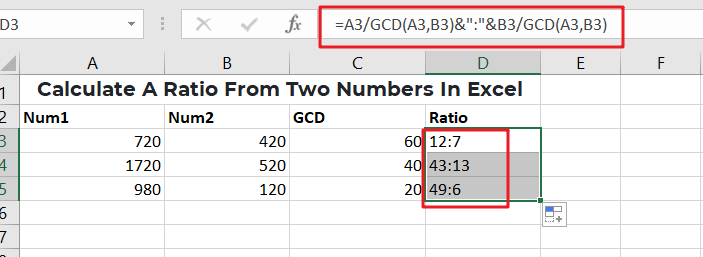## General Formula

In Ms Excel, use the formulae to compute the value of a bond on the issuance date.

` =num1/GCD(num1,num2)&":"&num2/GCD(num1,num2)`

## Explanation Of Syntax

You must be aware of the Formula’s syntax to use it to complete your task.

• GCD: The GCD Function computes the greatest common denominator of two or more numbers. We can then utilize GCD to calculate our ratios. The GCD Function is quite simple to use. Enter =GCD (followed by the integers you want to determine the greatest common divisor of) into the calculator (separated by commas).
• The comma symbol (,): this symbol acts as a separator that aids in the separation of a list of values.
• Parenthesis (): This symbol’s primary function is to group the elements.
• Division (/): This symbol divides values or integers.

## Summary

You may use division, the GCD function, and concatenation to get the ratio of two integers (e.g., 7:3, 11:9, etc.). The first number (the antecedent) is represented by num1 in the general version of the Formula (above), while the second number is represented by num2 (the consequent).

The active cell in the example has the following formula:

`=A3/GCD(A3,B3)&":"&B3/GCD(A3,B3)`It should be noted that the GCD function only works with integers.

## Explanation

This Formula appears hard, but it is pretty simple because it is composed of two parts:

`= (number1 formula) &":"& (formula for number2)`

The GCD function is used on the left to determine the greatest common divisor (GCD) of the two integers. The first number is then divided by the GCD. The identical processes are carried out with the second number on the right.

The results of the right and left procedures are then concatenated, with the colon (“:”) acting as a separator.

=GCD(A3,B3)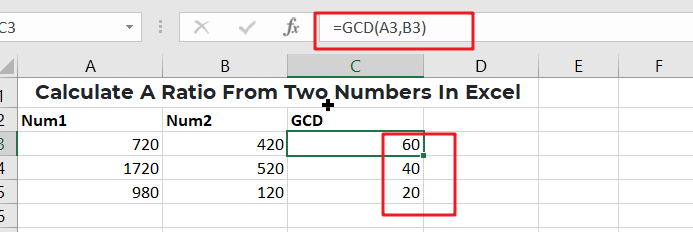=A3/GCD(A3,B3)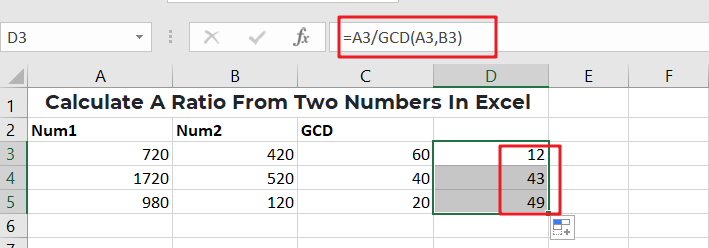=A3/GCD(A3,B3)&”:”&B3/GCD(A3,B3)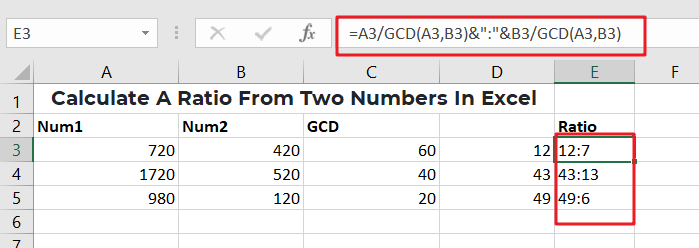It should be noted that the end outcome of this Formula is a text value.

If you’re still confused, don’t worry since both the following second and third examples will clear everything out for you and vanish all your confusion.

To compute a ratio from two values in Ms Excel, follow the instructions below.

For example, you would need to construct a sample data set in Ms Excel.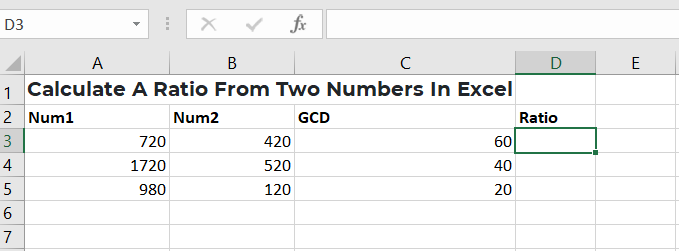Data samples

After that, enter the following Formula into the formula bar to get the ratio of two integers.

=A3/GCD(A3,B3)&”:”&B3/GCD(A3,B3)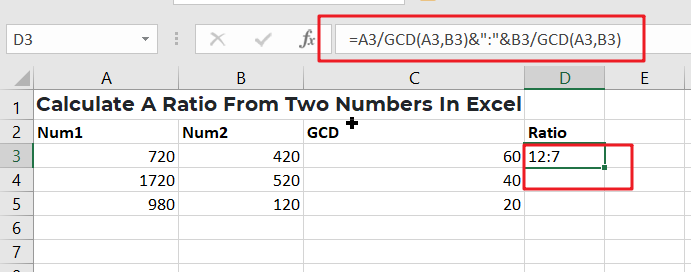press the Enter key to obtain the result in cell D3.

Finally, press Ctrl+ D to see the results in the other columns.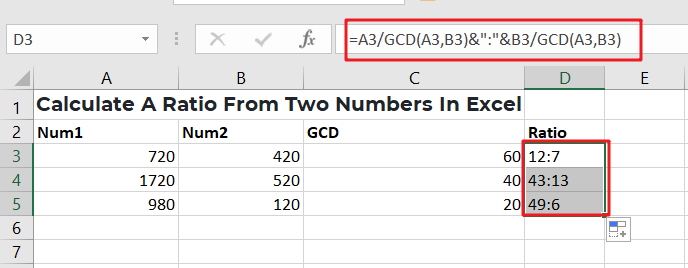For further understanding, let’s explore the third example;

Assume that we have the following two value lists in Ms Excel: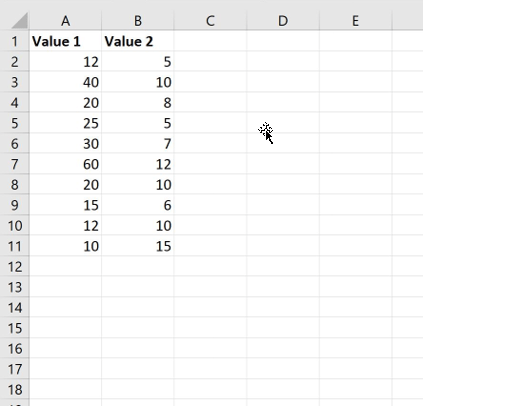Assume we want to compute the ratio of Value 1 to Value 2 in each row.

In cell C2, enter the following formula:

`=A2/GCD(A2, B2)&":"&B2/GCD(A2, B2)`

The screenshot below demonstrates how to apply this Formula in practice: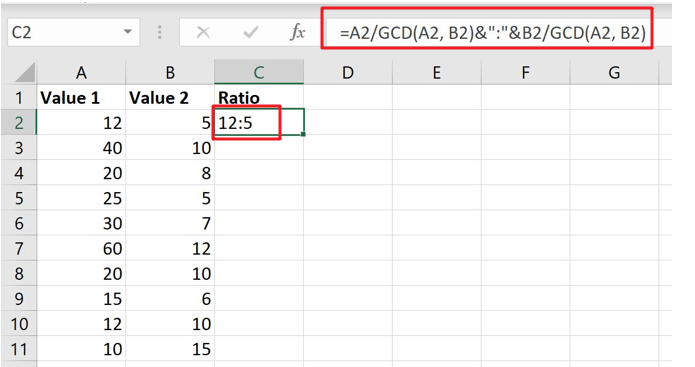The greatest common divisor of 12 and 5 is 1, as it turns out. As a result, when we divide each number by 1, we get a ratio of 12:5.

To determine the ratio for the two numbers in each row, we copy and paste this Formula in cell C2 down to every remaining cell in column C: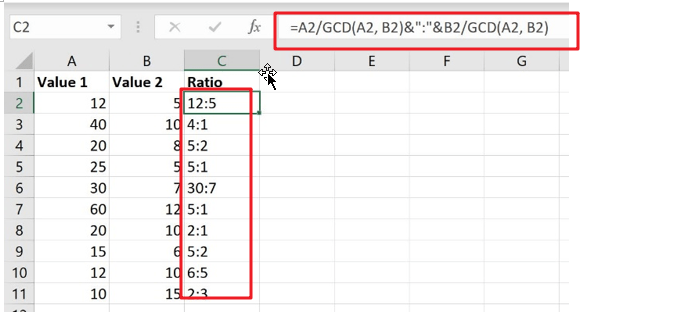## Conclusion

I hope you liked this tutorial on computing a ratio in Ms Excel from two data sets and that it helped you understand the entire procedure.

### Related Functions

• Excel GCD function
The Excel  GCD function Returns the greatest common divisor of two or more integers.The syntax of the GCD function is as below:= GCD(number1, [number2], …)